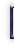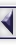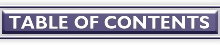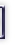## Taming rand() and random()

#### By Ken O. Burtch

In the lower levels of the Ontario Science Center in Toronto, Canada, there is a wide circular device made of thin rods of steel. Curious bystanders can take billiard balls, put there for that purpose, and let them loose on the machine.  The balls whiz along their rails, richocheting off pins, clanging through wind chimes, grabbed by counterweighted arms and lifted towards the ceiling.  At several places the balls chose one rail or another purely at random.  How is it that a construct not powered in any way, laid out in a rigid pattern, still produces unexpected results?

Writing programs that use random numbers requires an understanding of error estimation, probability theory, statistics and other advanced numeric disciplines.

Bunk.

Random numbers are about getting your programs to do the unexpected without a core dump being involved.  They're about having fun.

# Random But Not Really Random

Computers do not use "real world" random numbers.  Like the billiard-ball machine, computers are rigid, constrained by rules and logical behaviour.  For a computer to generate truly random numbers, it would have to choose numbers by examining real world events.  In the early days, people might roll some 10-sided dice and compose a list of digits for a program to use.

Unfortunately real-world random numbers can be unexpectedly biased.  As the old saying goes, "the real world is a special case."  Instead, computers rely on mathematics to generate uniformly distributed (that is, random but not too random) numbers.  They are "pseudo-random", generated by mathematic functions which create a seemingly non-repeating sequence.  Over time, the numbers in the sequence will reliably occur equally often, with no one number being favoured over another.

The Linux standard C library (stdlib.h) has two built-in random number functions.  The first, rand(), returns a random integer between 0 and RAND_MAX.  If we type

printf( " rand() is %d\n", rand() );
printf( " rand() is %d\n", rand() );
printf( " rand() is %d\n", rand() );

rand() will return values like

rand() is 1750354891
rand() is 2140807809
rand() is 1844326400

Each invocation will return a new, randomly chosen positive integer number.

The other standard library function, random(), returns a positive long integer.  On Linux, both integer and long integer numbers are the same size.  random() has some other properties that are discussed below.

There are also older, obsolete functions to produce random numbers

* drand48/erand48 return a random double between 0..1.
* lrand48/nrand48 return a random long between 0 and 2^31.
* mrand48/jrand48 return a signed random long.

These are provided for backward compatibility with other flavours of UNIX.

rand() and random() are, of course, totally useless as they appear and are rarely called directly.  It's not often we're looking for a number number between 0 and a really big number: the numbers need to apply to actually problems with specific ranges of alternatives.  To tame rand(), its value must be scaled to a more useful range such as between 1 and some specific maximum.  The modulus (%) operator works well: when a number is divided, the remainder is between 0 and 1 less than the original number.  Adding 1 to the modulus result gives the range we're looking for.

int rnd( int max ) {
return (rand() % max) + 1;
}

This one line function will return numbers between 1 and a specified maximum.  rnd(10) will return numbers between 1 and 10, rnd(50) will return numbers between 1 and 50.  Real life events can be simulated by assigning numbers for different outcomes.  Flipping a coin is rnd(2)==1 for heads, rnd(2)==2 for tails.  Rolling a pair of dice is rnd(6)+rnd(6).

The rand() discussion in the Linux manual recommends that you take the "upper bits" (that is, use division instead of modulus) because they tend to be more random.  However, the rnd() function above is suitably random for most applications.

The following test program generates 100 numbers between 1 and 10, counting how often each number comes up in the sequence.  If the numbers were perfectly uniform, they would appear 10 times each.

int graph;
int i;

for (i=1; i<=10; i++)
graph[i] = 0;
for (i=1; i<=100; i++)
graph[ rnd(10) ]++;
printf( "for rnd(), graph[1..10] is " );
for (i=1; i<=10; i++)
printf( "%d " , graph[i] );
printf( "\n" );

When we run this routine, we get the following:

for rnd(), graph[1..10] is 7 12 9 8 14 9 16 5 11 9

Linux's rand() function goes to great efforts to generate high-quality random numbers and therefore uses a significant amount of CPU time.  If you need to generate a lot mediocre quality random numbers quickly, you can use a function like this:

unsigned int seed = 0;

int fast_rnd( int max ) {
unsigned int offset = 12923;
unsigned int multiplier = 4079;

seed = seed * multiplier + offset;
return (int)(seed % max) + 1;
}

This function sacrifices accuracy for speed: it will produce random numbers not quite as mathematically uniform as rnd(), but it uses only a few short calculations.  Ideally, the offset and multiplier should be prime numbers so that fewer numbers will be favoured over others.

Replacing rnd with fast_rnd() in the test functions still gives a reasonable approximation of rand() with

for fast_rnd(), graph[1..10] is 11 4 4 1 8 8 5 7 6 5

# Controlling the Sequence

A seed is the initial value given to a random number generator to produce the first random number.  If you set the seed to a certain value, the sequence of numbers will always repeat, starting with the same number.  If you are writing a game, for example, you can set the seed to a specific value and use the fast_rnd() to position enemies in the same place each time without actually having to save any location information.

seed = room_number;
num_enemy = fast_rnd( 5 );
for ( enemy=1; enemy<=num_enemy; enemy++ ) {
enemy_type[enemy] = fast_rnd( 6 );
enemy_horizontal[enemy] = fast_rnd( 1024 );
enemy_vertical[enemy] = fast_rnd( 768 );
}

The seed for the Linux rand() function is set by srand(). For example,

srand( 4 );

will set the rand() seed to 4.

There are two ways to control the sequence with the other Linux function, random().  First, srandom(), like srand(), will set a seed for random().

Second, if you need greater precision, Linux provides two functions to control the speed and precision of random().  With initstate(), you can give random() both a seed and a buffer for keeping the intermediate function result.  The buffer can be 8, 32, 64, 128 or 256 bytes in size.  Larger buffers will give better random numbers but will take longer to calculate as a result.

char state;                 /* 256 byte buffer */
unsigned int seed = 1;         /* initial seed of 1 */

initstate( seed, state, 256 );
printf( "using a 256 byte state, we get %d\n", random() );
printf( "using a 256 byte state, we get %d\n", random() );
initstate( seed, state, 256 );
printf( "resetting the state, we get %d\n", random() );

gives

using a 256 byte state, we get 510644794
using a 256 byte state, we get 625058908
resetting the state, we get 510644794

You can switch random() states with setstate(), followed by srandom() to initialize the seed to a specific value.
setstate() always returns a pointer to the previous state.

oldstate = setstate( newstate );

Unless you change the seed when your program starts, your random numbers will always be the same.  To create changing random sequences, the seed should be set to some value outside of the program or users control. Using the time code returned by time.h's time() is a good choice.

srand( time( NULL ) );

Since the time is always changing, this will give your program a new sequence of random numbers each time it begins execution.

# Randomizing Lists

One of the classic gaming problems that seems to stump many people is shuffling, changing the order of items in a list.  While I was at university, the Computer Center there faced the task of sorting a list of names.  Their solution was to print out the names on paper, cut the paper with scissors, and pull the slips of paper from a bucket and retype them into the computer.

So what is the best approach to shuffling a list?  Cutting up a print out?  Dubious.  Exchanging random items a few thousand times?  Effective, but slow and it doesn't guarantee that all items will have a chance to be moved.  Instead, take each item in the list and exchange it with some other item.  For example, suppose we have a list of 52 playing cards represented by the numbers 0 to 51.  To shuffle the cards, we'd do the following:

int deck[ 52 ];
int newpos;
int savecard;
int i;

for ( i=0; i<52; i++ )
deck[i] = i;
printf( "Deck was " );
for ( i=0; i<52; i++ )
printf( "%d ", deck[i] );
printf( "\n" );
for ( i=0; i<52; i++ ) {
newpos = rnd(52)-1;
savecard = deck[i];
deck[i] = deck[newpos];
deck[newpos] = savecard;
}
printf( "Deck is " );
for ( i=0; i<52; i++ )
printf( "%d ", deck[i] );
printf( "\n" );

The results give us a before and after picture of the deck:

Deck was 0 1 2 3 4 5 6 7 8 9 10 11 12 13 14 15 16 17 18 19 20 21 22 23 24 25 26 27 28 29 30 31 32 33 34 35 36 37 38 39 40 41 42 43 44 45 46 47 48 49 50 51
Deck is 35 48 34 13 6 11 49 41 1 32 23 3 16 43 42 18 28 26 25 15 7 27 5 29 44 2 47 38 39 50 31 17 8 14 22 36 12 30 33 10 45 21 46 19 24 9 51 20 4 37 0 40

# Different Types of Randomness

People acquainted with statistics know that many of real life events do not happen with a uniform pattern.  The first major repair for a car, for example, might happen between 5 and 9 years of after purchase, but it might be most common around the 7th year.  Any year in the range is likely, but its most likely to be in the middle of the range.

Small unexpected events like these occur in a bell curve shape (called a normal distribution in statistics).  Creating random numbers that conform to such a complex shape may seem like a duanting task, but it really isn't.  Since our rnd() function already produces nicely uniform "unexpected" events, we don't need a statistics textbook formula to generate normally distributed random numbers.  All we need to do is call rnd() a few times and take the average, simulating a normal distribution.

int normal_rnd( int max ) {
return (rnd( max ) + rnd( max ) + rnd( max ) + rnd( max ) +
rnd( max ) + rnd( max ) + rnd( max ) + rnd( max ) ) / 8;

}

Using normal_rnd() in the test function, we get values that are clustered at the mid-point between 1 and max:

for normal_rnd(), graph[1..10] is 0 0 4 26 37 23 10 0 0 0

Normal random numbers can be used to make a game more life-like, making enemy behaviour less erratic.

For numbers skewed toward the low end of the range, we can create a low_rnd() which favours numbers near 1.

int low_rnd( int max ) {
int candidate;

candidate = rnd( max );
if ( rnd( 2 ) == 1 )
return candidate;
else if ( max > 1 )
return low_rnd( max / 2 );
else
return 1;
}

In each recursion, low_rnd() splits the range in half, favoring the lower half of the range.  By deducting a low random number from the top of the range, we could write a corresponding high_rnd() favoring numbers near the max:

int high_rnd( int max ) {
return max - low_rnd( max ) + 1;
}

The skewing is easily seen when using the test program:

for low_rnd(), graph[1..10] is 36 15 11 8 9 3 4 3 3 8
for high_rnd(), graph[1..10] is 4 5 8 5 4 10 6 10 14 34

# Random If Statements

Arbitrary branches in logic can be done with a odds() function.

int odds( int percent ) {
if ( percent <= 0 )
return 0;
else if ( percent > 100 )
return 1;
else if ( rnd( 100 ) <= percent )
return 1;
return 0;
}

This function is true the specified percentage of the time making it easy to incorporate into an if statement.

if ( odds( 50 ) )
printf( "The cave did not collapse!\n" )
else
printf( "Ouch! You are squashed beneath a mountain of boulders.\n" );

The standard C library rand() and random() functions provide a program with uniformly distributed random numbers.  The sequence and precision can be controlled by other library functions and the distribution of numbers can be altered by simple functions.  Random numbers can add unpredictability to a program and are, of course, the backbone to exciting play in computer games.

##### Copyright © 2001, Ken O. Burtch. Copying license http://www.linuxgazette.net/copying.html Published in Issue 63 of Linux Gazette, Mid-February (EXTRA) 2001